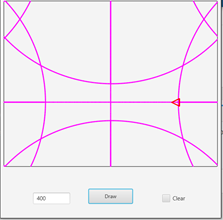# 🐢 B4XTurtle - Library for teachers and parents

#### inakigarm

##### Well-Known Member
Is it "normal" that if turtle draws outside canva's pane the results are like that?
If the radius is high, the turtle continue to draw from side's canvas (It seems that should be better not to draw outside the pane and not continue from the other sides of the canvas)Staff member

#### Erel

Staff member
Is it "normal" that if turtle draws outside canva's pane the results are like that?
Yes. The drawing continues on the other side. This is how classic Logo works.

#### Sandman

##### Well-Known Member
i am sure there is a better and shorter way
There might be a shorter way, but I can't see anything wrong with solving it like this. Looks good to me!

#### LucaMs

##### Expert
For i = 1 To 360 Turtle.MoveForward(((r*2)*cPI)/360).TurnLeft(1) Next
Why calculate 360 times the "fixed" value: (r*2)/cPI/360?#### LucaMs

##### Expert
i am sure there is a better and shorter way
I did it differently because I hoped to be able to make a continuous track, but I don't think it's possible; for this purpose I used the PushState and PopState methods, which allow that "effect".

I hope I haven't written too incomprehensible and illogical things ... I'm sleepy#### LucaMs

##### Expert
Just Circle:

B4X:
``````Public Const CLOCKWISE As Int = 1
Public Const COUNTERCLOCKWISE As Int = 2``````

B4X:
``````' Wise - pass one of the two contants provided:
' CLOCKWISE or COUNTERCLOCKWISE.
Sub Circle (x As Float, y As Float, r As Float, Wise As Int)
Turtle.SetX(x).SetY(y - r / 2)

If Wise = CLOCKWISE Then
Turtle.SetAngle(0)
Else
Turtle.SetAngle(180)
End If

Dim v As Float = (r * cPI) / 360
For i = 1 To 360
If Wise = CLOCKWISE Then
Turtle.MoveForward(v).TurnRight(1)
Else
Turtle.MoveForward(v).TurnRight(-1)
End If
Next
End Sub``````

•Erel

#### LucaMs

##### Expert
Just Circle:•Erel

#### Erel

Staff member
Why calculate 360 times the "fixed" value: (r*2)/cPI/360?
This is a preoptimization which is not relevant unless you plan to draw millions of circles per second (in rabbit mode).

•Johan Hormaza and ilan

#### LucaMs

##### Expert
This is a preoptimization which is not relevant unless you plan to draw millions of circles per second (in rabbit mode).
I know (and I could see it) but it is just a good practice avoid "logical errors".

#### ilan

##### Expert
Why calculate 360 times the "fixed" value: (r*2)/cPI/360?i also used a variable but when i posted the code i wanted it to be as short as possible. you know that's the "key" in a challengein real life i would use a variable and do the calculation only once.

Just Circle:

B4X:
``````Public Const CLOCKWISE As Int = 1
Public Const COUNTERCLOCKWISE As Int = 2``````

B4X:
``````' Wise - pass one of the two contants provided:
' CLOCKWISE or COUNTERCLOCKWISE.
Sub Circle (x As Float, y As Float, r As Float, Wise As Int)
Turtle.SetX(x).SetY(y - r / 2)

If Wise = CLOCKWISE Then
Turtle.SetAngle(0)
Else
Turtle.SetAngle(180)
End If

Dim v As Float = (r * cPI) / 360
For i = 1 To 360
If Wise = CLOCKWISE Then
Turtle.MoveForward(v).TurnRight(1)
Else
Turtle.MoveForward(v).TurnRight(-1)
End If
Next
End Sub``````

code is to LONG to do only a circle#### LucaMs

##### Expert
I don't like "compact code", I much prefer code that is easy to read, debug, maintain and upgrade.

#### LucaMs

##### Expert
code is to LONG to do only a circle
That code, as you see, places the turtle in a precise position with respect to the center and allows you to draw both clockwise and counterclockwise. Of course, if you remove the empty lines, it may seem shorter#### ilan

##### Expert
what about drawing a "fish" (our turtle) swimming on a wave?B4X:
``````Sub drawwave(r As Float)
...
End Sub``````

•inakigarm, Erel and LucaMs

#### ilan

##### Expert
wave height and length.

B4X:
``drawwave(60)``

#### ilan

##### Expert
no one with a solution?

#### LucaMs

##### Expert
no one with a solution?
I'm too sleepy. I would say two loops, the external one with a step which is then the limit of the internal loop.
Something like:
B4X:
``````For i = 0 to AAA Step BBB
For J = i to i + BBB - 1 ' <--- for the wave, sine curve``````

#### ilan

##### Expert
I'm too sleepy. I would say two loops, the external one with a step which is then the limit of the internal loop.
Something like:
B4X:
``````For i = 0 to AAA Step BBB
For J = i to i + BBB - 1``````

actually its very simple:

B4X:
``````Sub drawwave(r As Float)
Dim oppositedirection As Boolean = True
Turtle.TurnRight(30)
For i = 1 To 2000
If oppositedirection Then Turtle.MoveForward(((r*2)*cPI)/360).TurnLeft(1) Else Turtle.MoveForward(((r*2)*cPI)/360).TurnRight(1)
If i Mod 60 = 0 Then oppositedirection = Not(oppositedirection)
Next
End Sub``````

•Erel and LucaMs

#### LucaMs

##### Expert
(r*2)*cPI)/360
Again•ilan

Replies
41
Views
11K
Replies
2
Views
2K
Replies
14
Views
3K
Replies
20
Views
3K